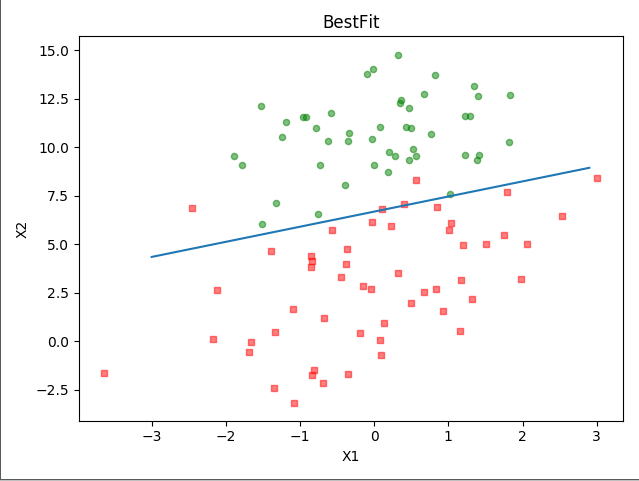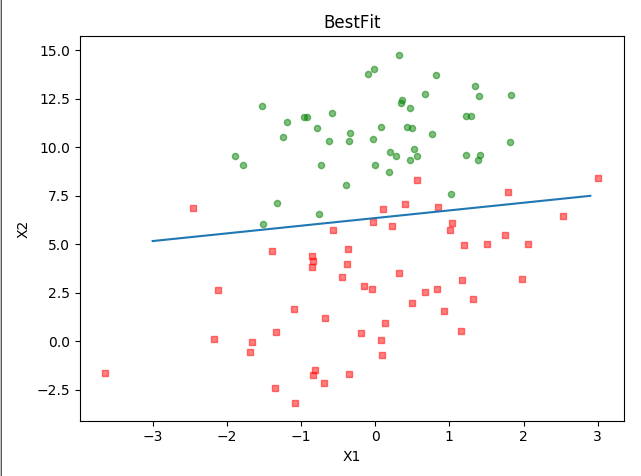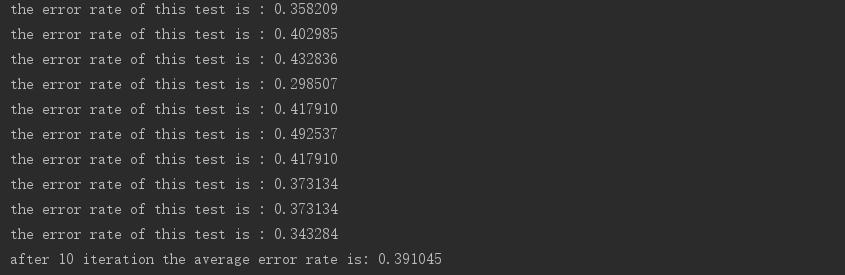Logistic回归的一般过程

1. 收集数据：采用任意方法收集数据。
2. 准备数据：由于需要进行距离计算，因此要求数据类型为数值型。另外，结构化数据格式则最佳。
3. 分析数据：采用任意方法对数据进行分析。
4. 训练算法：大部分时间将用于训练，训练的目的是为了找到最佳的分类回归系数。
5. 测试算法：一旦训练步骤完成，分类将会很快。
6. 使用算法：首先，我们需要输入一些数据，并将其转换成对应的结构化数值；接着，基于训练好的回归系数就可以对这些数值进行简单的回归计算，判定它们属于哪个类别；在这之后，我们就可以在输出的类别上做一些其他分析工作。

基于 Logistic 回归和 Sigmoid 函数的分类

Sigmoid函数

Sigmoid函数：$g(z) = \frac{1}{1+e^{-z}}$

Sigmoid函数是一种阶跃函数（step function）,当x为0时，Sigmoid函数值为0.5。随着x的增大，对应的Sigmoid值将逼近于1；而随着x的减小，Sigmoid值将逼近于0。如果横坐标刻度足够大， Sigmoid函数看起来很像一个阶跃函数。

最佳回归系数

Logistic回归是回归的一种方法，它利用的是Sigmoid函数阈值在[0,1]这个特性。Logistic回归进行分类的主要思想是：根据现有数据对分类边界线建立回归公式，以此进行分类。其实，Logistic本质上是一个基于条件概率的判别模型(Discriminative Model)。
Sigmoid函数的输入记为z：

$$z = w_0x_0+w_1x_1+w_2x_2+…+w_nx_n$$

hw(x)函数的值有特殊的含义，它表示结果取1的概率，因此对于输入x分类结果为类别1和类别0的概率分别为：

$$P(y=1|x;w) = h_w(x)$$

$$P(y=0|x;w) = 1-h_w(x)$$

$$Cost(h_w(x),y) = h_w(x)^y(1-h_w(x))^{1-y}$$

$$Cost(h_w(x),y) = ylogh_w(x) + (1-y)log(1-h_w(x))$$

$$J(w) = \sum_{i=1}^m[y^{(i)}logh_w(x^{(i)}) + (1-y)^{(i)}log(1-h_w(x^{(i)}))]$$

m为样本的总数，y(i)表示第i个样本的类别，x(i)表示第i个样本，需要注意的是w是多维向量，x(i)也是多维向量。

梯度上升法

$$∇f(x,y) = \begin{pmatrix} \frac{\partial{f(x,y)}}{\partial{x}}\ \frac{\partial{f(x,y)}}{\partial{y}} \end{pmatrix}$$

$$w:= w+\alpha∇_wf(w)$$

$$\frac{\partial{J(w)}}{\partial{w_j}} = \frac{\partial{J(w)}}{\partial{g(w^Tx)}} \frac{\partial{g(w^Tx)}}{\partial{w^Tx}} \frac{\partial{w^Tx}}{\partial{w_j}}$$

$$\frac{\partial{J(w)}}{\partial{g(w^Tx)}} = y\frac{1}{g(w^Tx)} + (y-1) \frac{1}{1-g(w^Tx)}$$

$$\frac{\partial{g(w^Tx)}}{\partial{w^Tx}} = g(w^Tx)(1-g(w^Tx))$$

$$\frac{\partial{w^Tx}}{\partial{w_j}} = \frac{\partial{J( w_0x_0+w_1x_1+w_2x_2+…+w_nx_n)}}{\partial{w_j}} = x_j$$

$$\frac{\partial{J(w)}}{\partial{w_j}} = (y-h_w(x))x_j$$

$$w_j:= w_j + \alpha\sum_{i=1}^m(y^{(i)}-h_w(x^{(i)}))x_j^{(i)}$$

训练算法

Logistic 回归梯度上升优化算法:

分析数据：画出决策边界

0是两个分类（类别1和类别0）的分界处。因此，我们设定 0 = w0x0 + w1x1 +w2x2，然后解出X2和X1的关系式（即分隔线的方程，注意X0＝1）。训练算法：随机梯度上升

1.alpha在每次迭代的时候都会调整，虽然alpha会随着迭代次数不断减小，但永远不会减小到0，这是因为公式中还存在一个常数项，必须这样做的原因是为了保证在多次迭代之后新数据仍然具有一定的影响。
2.这里通过随机选取样本来更新回归系数。这种方法将减少周期性的波动。
3.改进算法还增加了一个迭代次数作为第3个参数。如果该参数没有给定的话，算法将默认迭代150次。如果给定，那么算法将按照新的参数值进行迭代。从疝气病症预测病马的死亡率

测试算法：用 Logistic 回归进行分类

Logistic回归分类函数小结

Logistic回归的目的是寻找一个非线性函数Sigmoid的最佳拟合参数，求解过程可以由最优化算法来完成。在最优化算法中，最常用的就是梯度上升算法，而梯度上升算法又可以简化为随机梯度上升算法